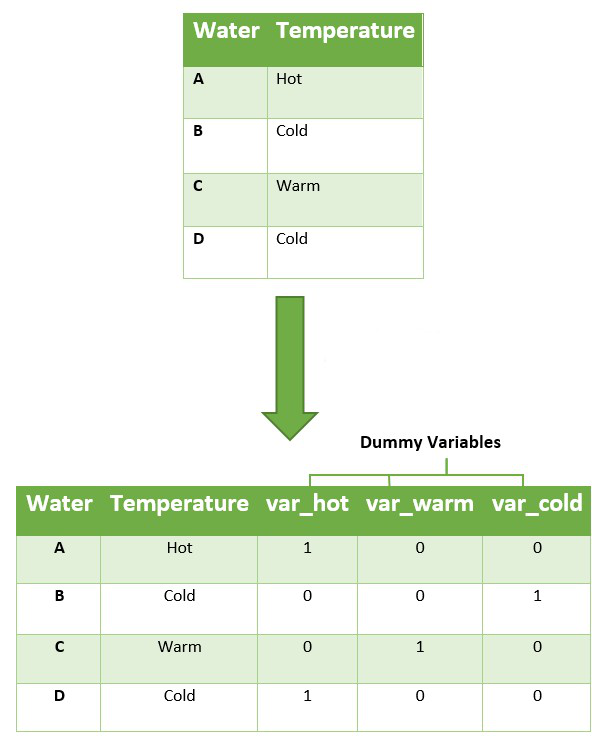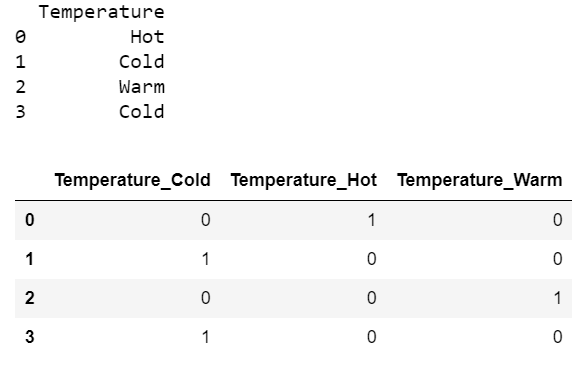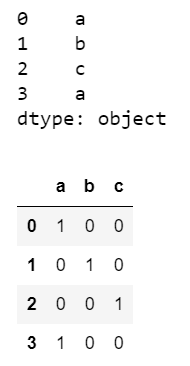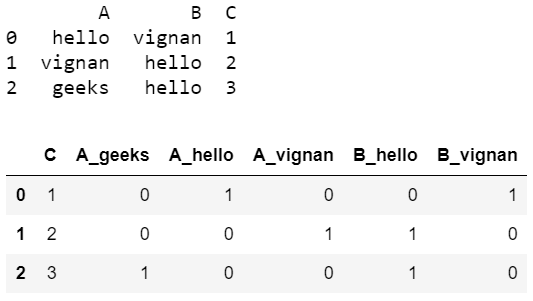# How to Create Dummy Variables in Python with Pandas?

• Last Updated : 08 Oct, 2021

A dataset may contain various type of values, sometimes it consists of categorical values. So, in-order to use those categorical value for programming efficiently we create dummy variables. A dummy variable is a binary variable that indicates whether a separate categorical variable takes on a specific value.

Explanation:

Attention geek! Strengthen your foundations with the Python Programming Foundation Course and learn the basics.

To begin with, your interview preparations Enhance your Data Structures concepts with the Python DS Course. And to begin with your Machine Learning Journey, join the Machine Learning - Basic Level CourseAs you can see three dummy variables are created for the three categorical values of the temperature attribute. We can create dummy variables in python using get_dummies() method.

Syntax: pandas.get_dummies(data, prefix=None, prefix_sep=’_’,)

Parameters:

• data= input data i.e. it includes pandas data frame. list . set . numpy arrays etc.
• prefix= Initial value
• prefix_sep= Data values separation.

Return Type: Dummy variables.

Step-by-step Approach:

• Import necessary modules
• Consider the data
• Perform operations on data to get dummies

Example 1:

## Python3

 `# import required modules``import` `pandas as pd``import` `numpy as np` `# create dataset``df ``=` `pd.DataFrame({``'Temperature'``: [``'Hot'``, ``'Cold'``, ``'Warm'``, ``'Cold'``],``                   ``})` `# display dataset``print``(df)` `# create dymmy variables``pd.get_dummies(df)`

Output:Example 2:

Consider List arrays to get dummies

## Python3

 `# import required modules``import` `pandas as pd``import` `numpy as np` `# create dataset``s ``=` `pd.Series(``list``(``'abca'``))` `# display dataset``print``(s)` `# create dymmy variables``pd.get_dummies(s)`

Output:Example 3:

Here is another example, to get dummy variables.

## Python3

 `# import required modules``import` `pandas as pd``import` `numpy as np` `# create dataset``df ``=` `pd.DataFrame({``'A'``: [``'hello'``, ``'vignan'``, ``'geeks'``],``                   ``'B'``: [``'vignan'``, ``'hello'``, ``'hello'``],``                   ``'C'``: [``1``, ``2``, ``3``]})` `# display dataset``print``(df)` `# create dymmy variables``pd.get_dummies(df)`

Output:My Personal Notes arrow_drop_up